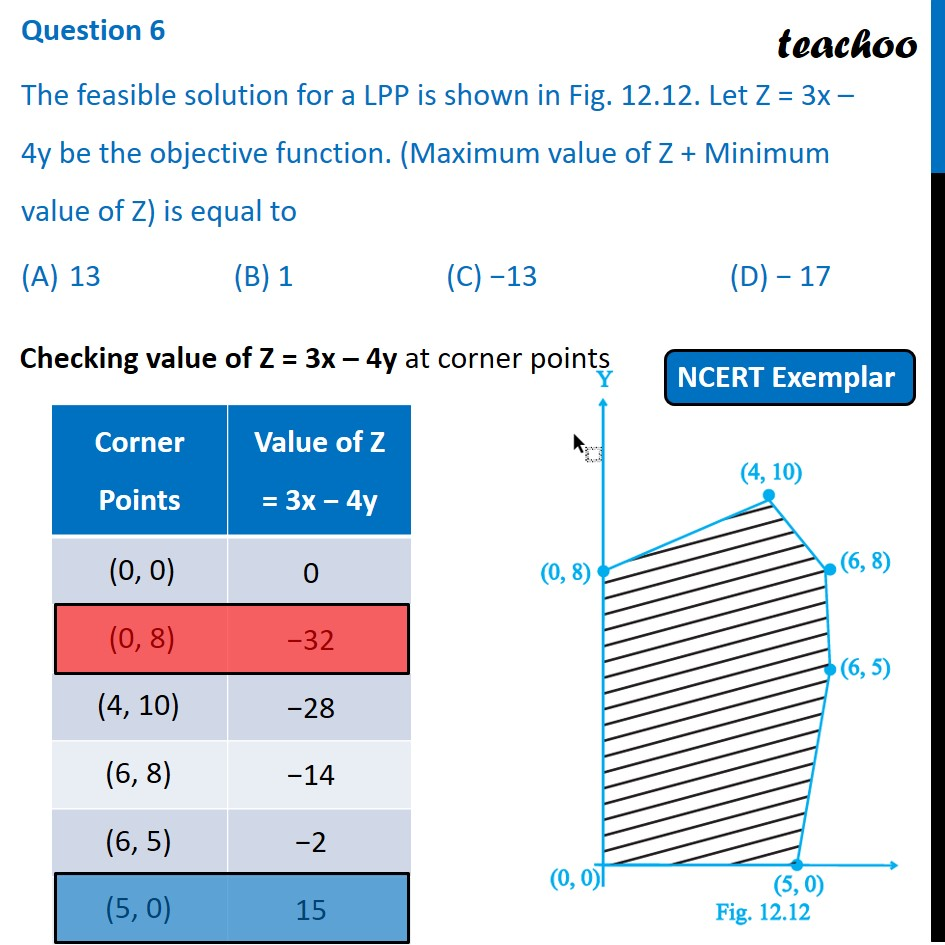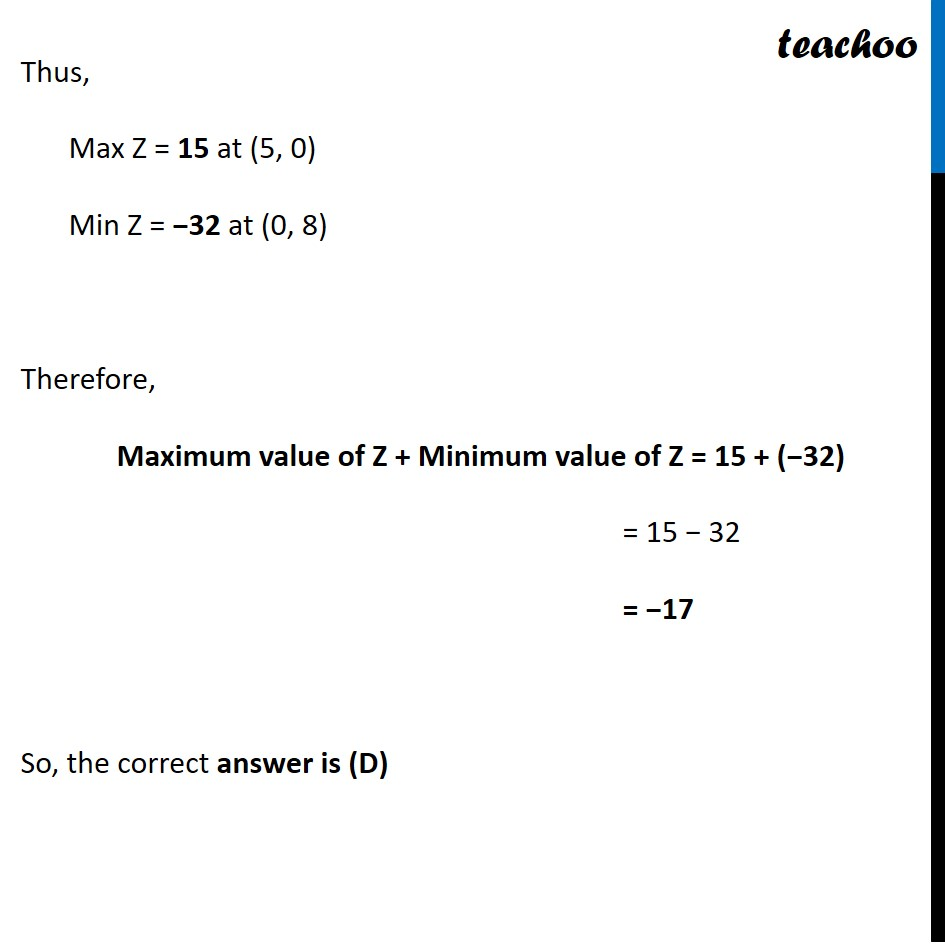NCERT Exemplar - MCQs

Chapter 12 Class 12 Linear Programming
Serial order wise

## (C) −13                        (D) − 17

This question is similar to Question 38 Choice 2 - Sample Paper 2021 Class 12 - Linear ProgrammingGet live Maths 1-on-1 Classs - Class 6 to 12

### Transcript

Question 6 The feasible solution for a LPP is shown in Fig. 12.12. Let Z = 3x – 4y be the objective function. (Maximum value of Z + Minimum value of Z) is equal to 13 (B) 1 (C) −13 (D) − 17 Checking value of Z = 3x – 4y at corner points Thus, Max Z = 15 at (5, 0) Min Z = −32 at (0, 8) Therefore, Maximum value of Z + Minimum value of Z = 15 + (−32) = 15 − 32 = −17 So, the correct answer is (D)